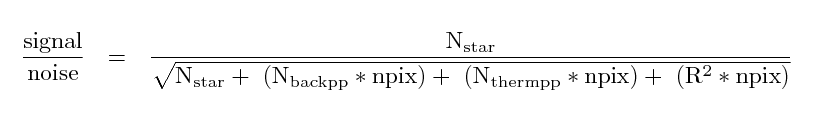### Signal-to-noise calculations in photometry

In short,• N(star) is the number of electrons from the star which fall within the aperture
• N(backpp) is the number of electrons Per Pixel
• N(thermpp) is the number of electrons Per Pixel
• R is the readout noise per pixel, in electrons
• npix is the number of pixels in the aperture